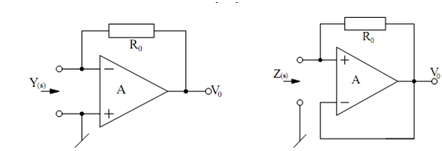## Realising Some Useful Functional Circuits Assignment Help

Assignment Help: >> Effect of Op-amp Pole - Realising Some Useful Functional Circuits

Realising Some Useful Functional Circuits Employing the Op-amp Pole:

Now we show how the op-amp pole, which is otherwise parasitic, may be used to advantage in realizing definite types of functional circuits. Assume first the circuits shown in Figure

Supposing the gain of the op-amp to be represented by Eq. a routine analysis of the circuit of Figure (a) yields its input admittance as

Y (s) = ( 1 /R0) +  A0 ωp /s R0

which represents a parallel-RL admittance with equivalent resistance equal to R-0 and equivalent inductance Leq equal to R0 / A0 ωp . Alternatively, a similar analysis of the circuit of the Figure (b) shows

Z (s) = R0  +( A0 ωp R0 )/ s

that represents a series-RC impedance with equivalent resistance equal to R0 and

equivalent capacitance Ceq equal to 1/A0 ωp RpFigure: (a) Simulated Inductor; and (b) Simulated Capacitor

Therefore, one may simulate the effects of an inductance and a capacitance just by using an op- amp and a resistor. These circuits may be utilized to advantage in many situations.